What is Area? How to measure it?

Measurement of area. Measuring area of regular and irregular shaped objects.

Learner's Guide

Recap:

In the previous session, we have learnt:

• Length, Time, Mass etc are fundamental quantity.
• Derived quantity is made from 2 or more than 2 fundamental quantities.
• Area, volume, density etc are derived quantity.

Motivation:

In this session, we will learn about a derived quantity: Area.

• What is area?
• How to measure area of ?
• regular shaped objects.
• irregular shaped object.

------------------------------------

What is area?

Which of the following a correct formulae of area?
1. Length
2. Length X Length
3. Length X Length X Length
4. Metre

Length X Length

Is width a fundamental quantity?

Yes.

Width is nothing but a type of length.

Other type of length:

1. distance
2. height

How to measure the area of regular shaped objects?

What is regular shaped object?

• A regular shaped object has predefined shape.
• Area of such shape are calculated using a specific formulae.
• This formulae remains applicable for all size.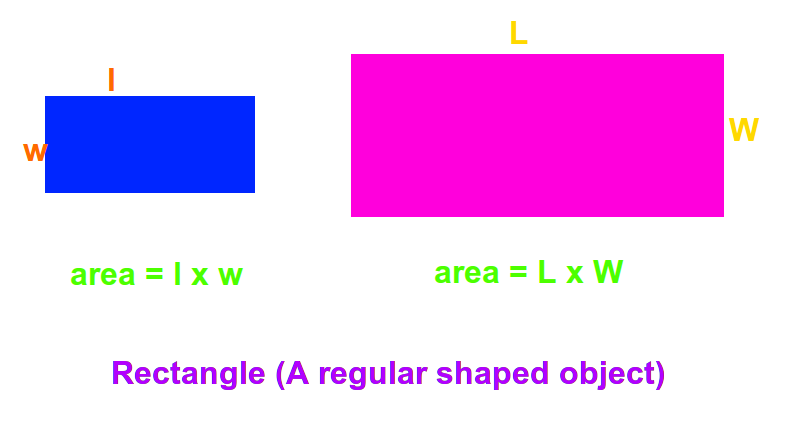What is the area in the below image if L= 5 metre and w = 4 metre?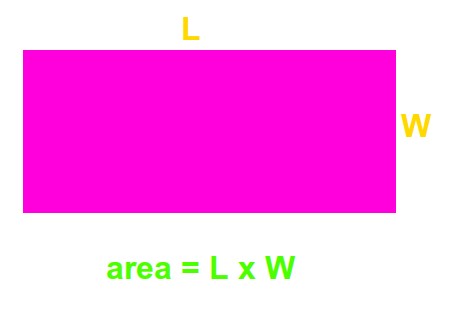area = L x W = 5 metre X 4 metre = 20 metre x metre = 20 metre² = 20 m²

The area of regularly shaped figures can be calculated using the relevant formulae.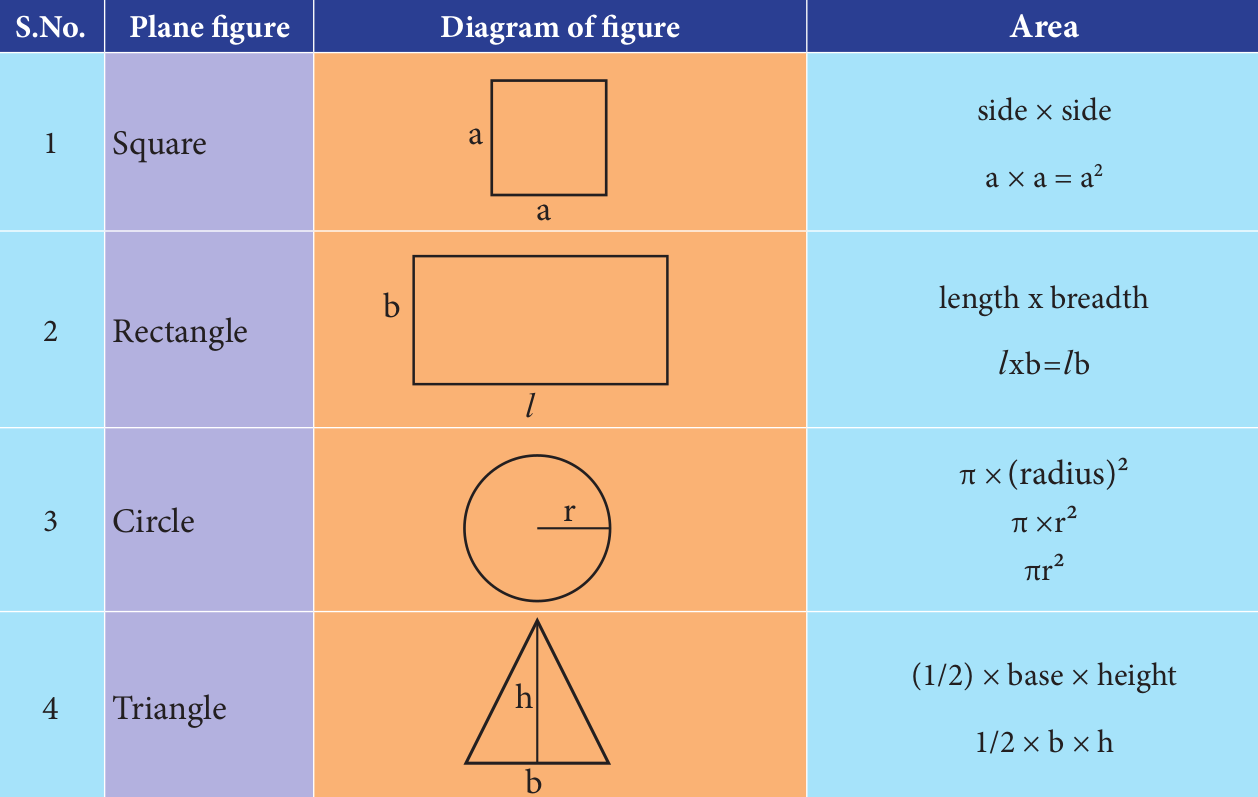What is the area of a circle if it's diameter is 6?
1. 36 π
2. 9 π
3. 6 π
4. 3 π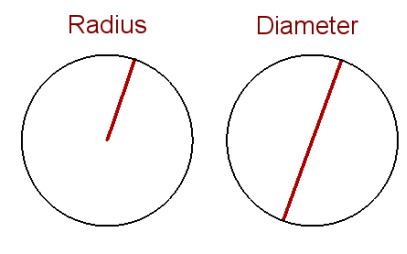Diameter = 2 x Radius

2 R = 6

⇒ R = 3

Area = π (R)²

⇒ Area = π (3)²

⇒ Area =  9 π

------------------------------------

How to measure the area of irregular shaped object?

What is the are of shaded region if each small box area is 1 m² ?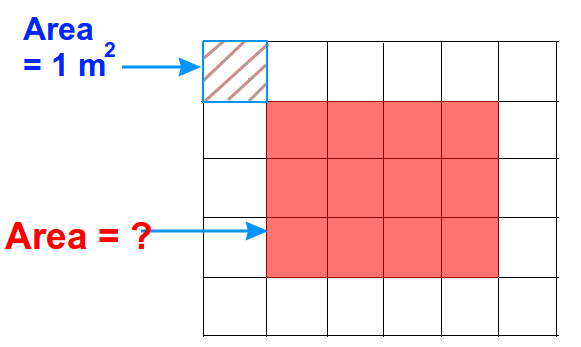We use similar technique in calculating area of irregular shaped objects

Watch the below video to learn in detail.

What is the area of shaded region if each small box area is 2 m²?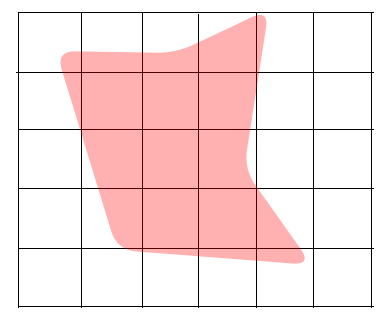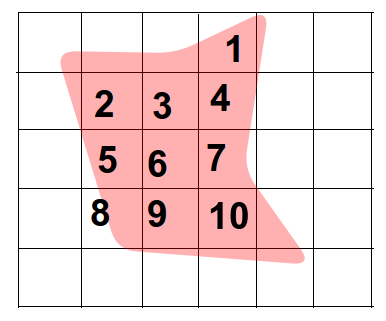Total squares inside the shaded region = 10

Area of shaded region = 10 x 2 m²

⇒ Area of shaded region = 20 m²

Exercise

Tip: Think before answering.

• In general, how many lengths needs to be measured to measure the area?

1. 2
2. 3
3. 5
4. 1
Difficulty Level: Easy

2

• Area of irregular shaped object can not be measured.

True or False?

Difficulty Level: Easy

False.

• If a rectangle has length = 5 cm, and it has breadth = 5

What is the area?

1. 10
2. 25
3. 32
4. 30
Difficulty Level: Easy

25

Area of rectangle = Length x Breadth

= 5 x 5

= 25

• Count the valid squares inside both the images: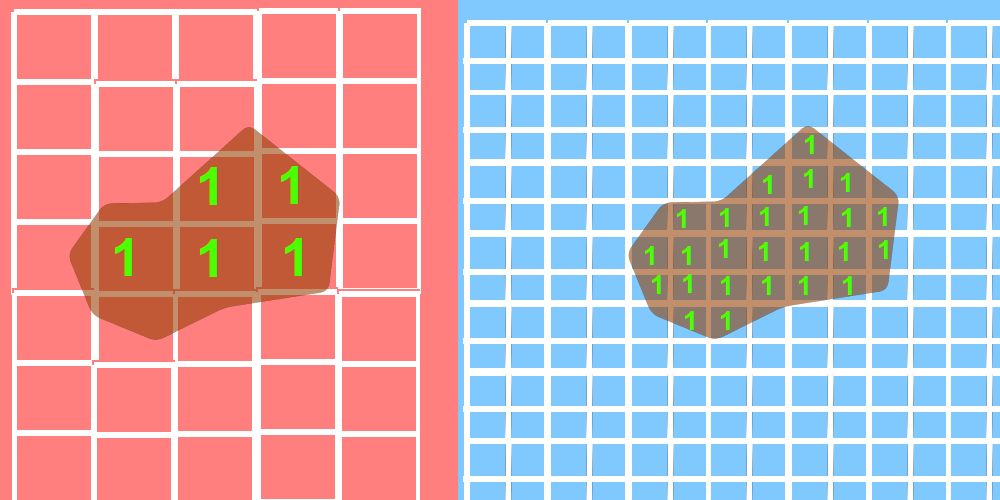Difficulty Level: Hard

Number of squares inside the irregular shape:

• Image 1 = 5 squares
• Image 2 = 25 squares

area of 1 square on left = 1 m²

area of 1 square on right = 1/4  m²

Area of left side irregular shape = area of 5 big squares = 5 m²

Area of left side irregular shape = area of 25 small squares = 25/4 m² = 6.25 m²

Conclusion:

As we decrease the area of mesh, we get more approximate result.

• Area of circle has different unit than area of square.

True or False?

Difficulty Level: Moderate

False.

SI Unit of area is (m²) is same for all the areas.